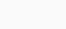INTRODUCTION

Calculus relies heavily on the derivative concept. Mathematicians and applied mathematicians use it in all fields. Researchers and engineers in other fields have also accepted this concept.

There are many applications of differentiation in many fields of science. For this reason, it is important to have good methods for computing and manipulating derivatives. School taught you the basic rules of differentiation – symbolic methods suitable for pencil-and-paper calculations. The most common programming environments do not support symbolic computations, so such methods have limited value on computers. There is also the problem of knowing a function at only a few isolated points in most practical applications. Numerical differentiation methods are derived by evaluating a function at a few points, finding the polynomial that interpolates the function at these points, and using the derivative of this polynomial to approximate the derivative. Using this technique, we can also track the so-called truncation error, which occurs when we differentiate a polynomial instead of the actual function. As well as truncation errors, round-off errors are inevitable when we use floating point numbers to calculate real numbers. It turns out that numerical differentiation is very sensitive to round-off errors, but these errors are quite easy to analyze.

The first question that comes to mind is: why do we need to approximate derivatives at all? Every function can be analytically differentiated, after all. Although we still need to approximate derivatives, there are several reasons for this: • Even if there is an underlying function that needs to be differentiated, we might know the values of the function only at a sampled data set. In some cases, we may think that the data set we have is all that exists and that there is no underlying function. It may still be worthwhile to study changes in data, which are related to derivatives, of course. In some cases, exact formulas are available, but they are very complicated, requiring a lot of function evaluations in order to compute the exact derivative. Instead of computing its exact value, it might be easier to approximate the derivative. Ordinary (or partial) differential equations are typically approximated as discrete approximations defined on a grid when solving them. As we have to evaluate derivatives at grid points, we must come up with methods for approximating derivatives at grid points using only lattice values. It is unknown what the underlying function is (in this case, the equation’s solution).Why numerical differential

Differentiation is one of the most important concepts in calculus, which has been used almost everywhere in many fields of mathematics and applied mathematics. It is natural that numerical differentiation should be an important technique for the engineers.

Limits

Engineers seem to have difficulty with this because they need to understand the algorithm according to the original problem. The numerical differentiation problem is ill-posed, causing this disadvantage. Several methods have been proposed to overcome this difficulty, such as Tikhonov regularization method 3, mollification method 6, etc.

Numerical differentiation can be approached in several ways. Engineers use finite difference as the most common and simplest method. The disadvantage of this method is that, if the data contains errors, even if we have a lot of data, we can’t use it all. To put it another way, the grid size cannot be too small.

Applications

Detecting discontinuity curves from irregularly sampled data using numerical differentiation formulas. We present a method for detecting discontinuity curves, called faults, in scattered data. In the first step, a subset of points near the fault is extracted from the data set. Derivatives need to be computed numerically in many applications. The simplest approach simply uses the definition of the derivativefor some small numerical value ofConclusion

With this we can see the importance of Numerical differentiation in terms of calculus in math. This was just the introduction about it. further we will discuss about it more in detail. Thank you for your time and see you soon.